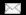Full Professor, Colorado State UniversityWINNER'S CURSE: Prototype Web Bidding System SAS Statements SAS Results SAS Experimental Design Alternative Model SAS statements TEST 3 SAS TEST_3_OUTPUT Main SAS Statements Group Incentive System Mid=1 and Intercept=theory Mid=1 Intercept=theory returnMain SAS File ```options nocenter; data us; infile 'd:\projects\cva\sas\total.dat' dlm=','; input market grpsize sigsize xprnc session period entity cv bid haven win profit; /* changing mistakes in bid entry (from experiment notes) */ if (market=7 and grpsize=5 and sigsize=5 and xprnc=2 and session=2 and entity=0 and cv=13052) then bid=12500; if (market=7 and grpsize=5 and sigsize=5 and xprnc=2 and session=2 and entity=0 and cv=13052) then win=0; if (market=7 and grpsize=5 and sigsize=5 and xprnc=2 and session=2 and entity=4 and cv=13052) then win=1; if (market=7 and grpsize=5 and sigsize=5 and xprnc=2 and session=2 and entity=4 and cv=13052) then profit=152; if (market=7 and grpsize=5 and sigsize=5 and xprnc=3 and session=2 and entity=3 and cv=9803) then bid=9341; if (market=7 and grpsize=5 and sigsize=5 and xprnc=3 and session=2 and entity=3 and cv=9803) then win=0; if (market=7 and grpsize=5 and sigsize=5 and xprnc=3 and session=2 and entity=1 and cv=9803) then win=1; if (market=7 and grpsize=5 and sigsize=5 and xprnc=3 and session=2 and entity=1 and cv=9803) then profit=53; if (market=7 and grpsize=5 and sigsize=1 and xprnc=3 and session=1 and entity=0 and cv=18361) then bid=18301; if (market=7 and grpsize=5 and sigsize=1 and xprnc=3 and session=1 and entity=0 and cv=18361) then win=0; if (market=7 and grpsize=5 and sigsize=1 and xprnc=3 and session=1 and entity=4 and cv=18361) then win=1; if (market=7 and grpsize=5 and sigsize=1 and xprnc=3 and session=1 and entity=4 and cv=18361) then profit=580; /* deleting obvious mistakes in bid entry (from experiment notes) */ if (market=3 and grpsize=1 and sigsize=5 and xprnc=2 and session=5 and entity=0 and cv=11545) then delete; if (market=3 and grpsize=1 and sigsize=5 and xprnc=2 and session=5 and entity=2 and cv=12975) then delete; if (market=3 and grpsize=1 and sigsize=5 and xprnc=2 and session=5 and entity=2 and cv=21027) then delete; if (market=5 and grpsize=5 and sigsize=5 and xprnc=2 and session=1 and entity=3 and cv=21027) then delete; if (market=5 and grpsize=5 and sigsize=5 and xprnc=2 and session=1 and entity=3 and cv=13641) then delete; if (market=5 and grpsize=5 and sigsize=5 and xprnc=2 and session=1 and entity=3 and cv=21027) then delete; if (market=5 and grpsize=5 and sigsize=5 and xprnc=2 and session=1 and entity=3 and cv=13641) then delete; if (market=7 and grpsize=1 and sigsize=1 and xprnc=2 and session=2 and entity=5 and cv=4577) then delete; if (market=7 and grpsize=1 and sigsize=1 and xprnc=2 and session=2 and entity=5 and cv=6074) then delete; if (market=7 and grpsize=1 and sigsize=5 and xprnc=2 and session=2 and entity=2 and cv=7881) then delete; if (market=7 and grpsize=1 and sigsize=5 and xprnc=2 and session=2 and entity=2 and cv=11183) then delete; if (market=7 and grpsize=1 and sigsize=5 and xprnc=2 and session=2 and entity=2 and cv=16188) then delete; if (market=7 and grpsize=1 and sigsize=5 and xprnc=2 and session=2 and entity=2 and cv=13065) then delete; if (market=7 and grpsize=1 and sigsize=5 and xprnc=2 and session=2 and entity=2 and cv=15257) then delete; if (market=7 and grpsize=1 and sigsize=5 and xprnc=2 and session=2 and entity=2 and cv=17801) then delete; if (market=7 and grpsize=1 and sigsize=5 and xprnc=2 and session=4 and entity=0 and cv=19552) then delete; if (market=7 and grpsize=1 and sigsize=5 and xprnc=2 and session=4 and entity=6 and cv=8575) then delete; if (market=7 and grpsize=5 and sigsize=5 and xprnc=2 and session=1 and entity=3 and cv=13319) then delete; if (market=7 and grpsize=5 and sigsize=5 and xprnc=2 and session=1 and entity=5 and cv=7027) then delete; /* if (market= and grpsize= and sigsize= and xprnc=2 and session= and entity= and period> ) then delete; */ matcher=entity*100000+cv; proc sort; by matcher; data signals; infile 'd:\projects\cva\sas\signals.dat' dlm=','; input entity cv high low signal; matcher=entity*100000+cv; proc sort; by matcher; data main; merge us signals; by matcher; /* if signal > 22500 then signal = 22500; if signal < 2500 then signal = 2500; */ if sigsize = 1 then high = signal; if sigsize = 1 then low = signal; theta=1800; mid=high-((high-low)/2); range=high-low; if sigsize = 5 and market = 3 then discount = ((theta - (range/2))/2); if sigsize = 5 and market = 5 then discount = (((theta - (range/2))/3)*2); if sigsize = 5 and market = 7 then discount = (((theta - (range/2))/4)*3); if sigsize = 1 and market = 3 then discount = 900; if sigsize = 1 and market = 5 then discount = 1200; if sigsize = 1 and market = 7 then discount = 1350; if sigsize = 5 and market = 3 then bid_y = bid - (range/4); if sigsize = 5 and market = 5 then bid_y = bid - (range/3); if sigsize = 5 and market = 7 then bid_y = bid - (3*range/8); if sigsize = 1 and market = 3 then bid_y = bid; if sigsize = 1 and market = 5 then bid_y = bid; if sigsize = 1 and market = 7 then bid_y = bid; predict = mid - discount; diff=bid-predict; vet_diff=bid-mid; country=0; ```CIS Dept |  College Of Business |  Colorado State University |  Aviation |  Home Page
 Voice: 970.491.7511016 Rockwell Hall - Colorado State University - Fort Collins, CO 80523Fax: 970.491.5205